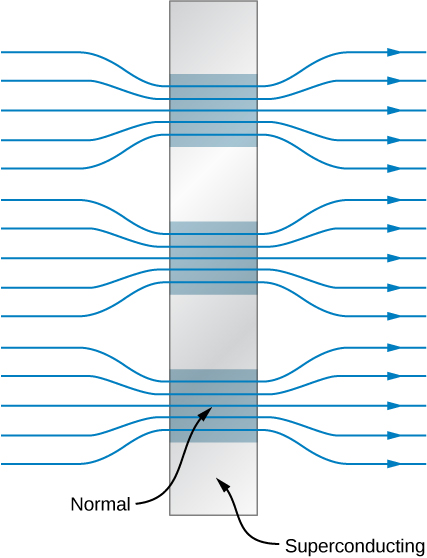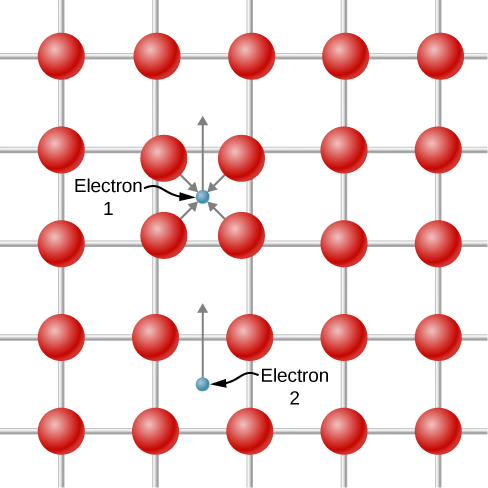# 9.8 Superconductivity  (Page 3/12)

 Page 3 / 12A schematic representation of the mixed state of a type II superconductor. Superconductors (the gray squares) expel magnetic fields in their vicinity.

## Niobium wire

In an experiment, a niobium (Nb) wire of radius 0.25 mm is immersed in liquid helium ( $T=4.2\phantom{\rule{0.2em}{0ex}}\text{K}$ ) and required to carry a current of 300 A. Does the wire remain superconducting?

## Strategy

The applied magnetic field can be determined from the radius of the wire and current. The critical magnetic field can be determined from [link] , the properties of the superconductor, and the temperature. If the applied magnetic field is greater than the critical field, then superconductivity in the Nb wire is destroyed.

## Solution

At $T=4.2\phantom{\rule{0.2em}{0ex}}\text{K},$ the critical field for Nb is, from [link] and [link] ,

${B}_{\text{c}}\left(4.2\phantom{\rule{0.2em}{0ex}}\text{K}\right)={B}_{\text{c}}\left(0\right)\left[1-{\left(\frac{4.2\phantom{\rule{0.2em}{0ex}}\text{K}}{9.3\phantom{\rule{0.2em}{0ex}}\text{K}}\right)}^{2}\right]=\left(0.20\phantom{\rule{0.2em}{0ex}}\text{T}\right)\left(0.80\right)=0.16\phantom{\rule{0.2em}{0ex}}\text{T.}$

In an earlier chapter, we learned the magnetic field inside a current-carrying wire of radius a is given by

$B=\frac{{\mu }_{0}I}{2\pi a},$

where r is the distance from the central axis of the wire. Thus, the field at the surface of the wire is $\frac{{\mu }_{0}{I}_{r}}{2\pi a}.$ For the niobium wire, this field is

$B=\frac{\left(4\pi \phantom{\rule{0.2em}{0ex}}×\phantom{\rule{0.2em}{0ex}}{10}^{-7}\phantom{\rule{0.2em}{0ex}}\text{T m/A}\right)\left(300\phantom{\rule{0.2em}{0ex}}\text{A}\right)}{2\text{π}\left(2.5\phantom{\rule{0.2em}{0ex}}×\phantom{\rule{0.2em}{0ex}}{10}^{-4}\phantom{\rule{0.2em}{0ex}}\text{m}\right)}=0.24\phantom{\rule{0.2em}{0ex}}\text{T}\text{.}$

Since this exceeds the critical 0.16 T, the wire does not remain superconducting.

## Significance

Superconductivity requires low temperatures and low magnetic fields. These simultaneous conditions are met less easily for Nb than for many other metals. For example, aluminum superconducts at temperatures 7 times lower and magnetic fields 18 times lower.

Check Your Understanding What conditions are necessary for superconductivity?

a low temperature and low magnetic field

## Theory of superconductors

A successful theory of superconductivity was developed in the 1950s by John Bardeen , Leon Cooper , and J. Robert Schrieffer , for which they received the Nobel Prize in 1972. This theory is known as the BCS theory    . BCS theory is complex, so we summarize it qualitatively below.

In a normal conductor, the electrical properties of the material are due to the most energetic electrons near the Fermi energy. In 1956, Cooper showed that if there is any attractive interaction between two electrons at the Fermi level, then the electrons can form a bound state in which their total energy is less than $2{E}_{\text{F}}$ . Two such electrons are known as a Cooper pair    .

It is hard to imagine two electrons attracting each other, since they have like charge and should repel. However, the proposed interaction occurs only in the context of an atomic lattice. A depiction of the attraction is shown in [link] . Electron 1 slightly displaces the positively charged atomic nuclei toward itself as it travels past because of the Coulomb attraction. Electron 2 “sees” a region with a higher density of positive charge relative to the surroundings and is therefore attracted into this region and, therefore indirectly, to electron 1. Because of the exclusion principle, the two electrons of a Cooper pair must have opposite spin.A Cooper pair can form as a result of the displacement of positive atomic nuclei. Electron 1 slightly displaces the positively charged atomic nuclei toward itself as it travels past because of the Coulomb attraction. Electron 2 “sees” a region with a higher density of positive charge relative to the surroundings and is therefore attracted into this region.

what is electro statics
when you consider systems consisting of fixed charges
Sherly
Diagram of the derive rotational analog equation of v= u+at
what is carat
what is chemistry
what chemistry ?
Abakar
where are the mcq
ok
Giorgi
acids and bases
Navya
How does unpolarized light have electric vector randomly oriented in all directions.
unpolarized light refers to a wave collection which has an equal distribution of electric field orientations for all directions
pro
In a grating, the angle of diffraction for second order maximum is 30°.When light of wavelength 5*10^-10cm is used. Calculate the number of lines per cm of the grating.
state the law of gravity 6
what is cathodic protection
its just a technique used for the protection of a metal from corrosion by making it cathode of an electrochemical cell.
akif
what is interferometer
what is interferometer
Abakar
Show that n1Sino1=n2Sino2
what's propagation
is it in context of waves?
Edgar
It is the manner of motion of the energy whether mechanical(requiring elastic medium)or electromagnetic(non interference with medium)
Edgar
determine displacement cat any time t for a body of mass 2kg under a time varrying force ft=bt³+csinkt
A round diaphragm S with diameter of d = 0.05 is used as light source in Michelson interferometer shown on the picture. The diaphragm is illuminated by parallel beam of monochromatic light with wavelength of λ = 0.6 μm. The distances are A B = 30, A C = 10 . The interference picture is in the form of concentric circles and is observed on the screen placed in the focal plane of the lens. Estimate the number of interference rings m observed near the main diffractive maximum.
A Pb wire wound in a tight solenoid of diameter of 4.0 mm is cooled to a temperature of 5.0 K. The wire is connected in series with a 50-Ωresistor and a variable source of emf. As the emf is increased, what value does it have when the superconductivity of the wire is destroyed?ByByBy RhodesBy